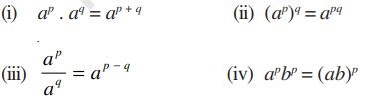Number System chapter of Class 9 Maths NCERT Book can be downloaded in PDF format from here. We have provided here the latest edition of the chapter along with precise NCERT Solutions.

Class 9 Maths Chapter 1 ´Number System` of NCERT Book is best to understand the concept of numbers and make your basics stronger for obtaining good results in exams. You will get here the latest edition of the chapter that will help you learn the updated information and prepare well for the examinations to be conducted in the current academic year 2021-2022.

About Class 9  Maths Chapter 1 Number System

Number System chapter explains the concept of rational and irrational numbers. You will get to learn representation of these forms of numbers along with special properties and identities. Some solved examples and exercise questions are given in the chapter for more clarification of concepts and also for self-assessment.

Major topics discussed in the chapter are:

→ Introduction to Number System

→ Irrational Numbers

→ Real Numbers and their Decimal Expansions

→ Representing Real Numbers on the Number Line

→ Operations on Real Numbers

Some important points to revise from the chapter are:

• A number r is called a rational number, if it can be written in the form p/q , where p and q are integers and q ≠ 0. 2.

• A number s is called a irrational number, if it cannot be written in the form p/q , where p and q are integers and q ≠ 0.

• The decimal expansion of a rational number is either terminating or non-terminating recurring.

• The decimal expansion of an irrational number is non-terminating non-recurring.

• All the rational and irrational numbers make up the collection of real numbers.

• If r is rational and s is irrational, then r + s and r – s are irrational numbers, and rs and r/s are irrational numbers, r ≠ 0. 8.

• positive real numbers a and b, the following identities hold:

• Let a > 0 be a real number and p and q be rational numbers. ThenAlso, check the NCERT Solutions for Class 9 Maths Chapter 5 from the following link:

Also Check:

NCERT Books for Class 9 (Latest Editions for 2021-22)

NCERT Solutions for Class 9 (Updated for 2021-22)